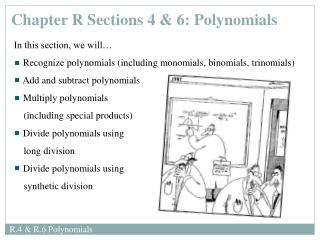DownloadDownload PresentationChapter R Sections 4 & 6: Polynomials

# Chapter R Sections 4 & 6: Polynomials

Télécharger la présentation## Chapter R Sections 4 & 6: Polynomials

- - - - - - - - - - - - - - - - - - - - - - - - - - - E N D - - - - - - - - - - - - - - - - - - - - - - - - - - -
##### Presentation Transcript

1. Chapter R Sections 4 & 6: Polynomials • In this section, we will… • Recognize polynomials (including monomials, binomials, trinomials) • Add and subtract polynomials • Multiply polynomials • (including special products) • Divide polynomials using • long division • Divide polynomials using • synthetic division

2. A polynomial in one variable is an algebraic expression of the form where are called the coefficients of the polynomial; n 0 is an integer and x is a variable. If , it is called the leading coefficient, and n is called the degree of the polynomial. Example: degree coefficients In other words, a polynomial is the sum of terms whose variables have whole number exponents. No variable appears in the denominator. R.4 & R.6 Polynomials: Recognize Polynomials

3. A polynomial with one term is called a monomial • A polynomial with two terms is called a binomial • A polynomial with three terms is called a trinomial R.4 & R.6 Polynomials: Recognize Polynomials

4. To add and subtract polynomials – combine like terms Examples: Perform the indicated operations. Express your answer as a single polynomial in standard form. R.4 & R.6 Polynomials: Add and Subtract Polynomials

5. To multiply polynomials – use the Distributive Property and the Laws of Exponents Examples: Perform the indicated operations. Express your answer as a single polynomial in standard form. R.4 & R.6 Polynomials: Multiplying Polynomials

6. R.4 & R.6 Polynomials: Multiplying Polynomials

7. Examples: Perform the indicated operations. Express your answer as a single polynomial in standard form. This is the difference of two squares This is a perfect square Note: We CANNOT distribute the exponent here! R.4 & R.6 Polynomials: Multiplying Polynomials

8. Examples: Perform the indicated operations. Express your answer as a single polynomial in standard form. This is the sum of two cubes This is a perfect cube R.4 & R.6 Polynomials: Multiplying Polynomials

9. Example: Divide using long division with integers. answer: Is 5 a factor of 82? Explain. check: quotient divisor dividend To check your result: (quotient) (divisor) + remainder = dividend R.4 & R.6 Polynomials: Divide Polynomials Using Long Division

10. Example: Find the quotient and the remainder when and check your result. • Write the division in long-division form • Ensure that each polynomial is in descending order • Insert a placeholder for missing terms (e.g. leave a space, 0x) R.4 & R.6 Polynomials: Divide Polynomials Using Long Division

11. Example:Find the quotient and the remainder when and check your result. check R.4 & R.6 Polynomials: Divide Polynomials Using Long Division

12. Example: Find the quotient and the remainder when and check your result. check R.4 & R.6 Polynomials: Divide Polynomials Using Long Division

13. We can find the quotient and remainder when a polynomial is divided by another polynomial of the form using a faster algorithm called synthetic division (it is a short-hand version of long division). Let’s re-do our first example where we used long division… • Ensure that each polynomial is in descending order • Write the division in synthetic-division form • Insert missing terms (e.g. 0x) R.4 & R.6 Polynomials: Divide Polynomials Using Synthetic Division

14. Example: Find the quotient and the remainder when and check your result. check Abducted by an alien circus company, Professor Cordes is forced to divide polynomials center ring. R.4 & R.6 Polynomials: Divide Polynomials Using Synthetic Division

15. Example: Find the quotient and the remainder when and check your result. check R.4 & R.6 Polynomials: Divide Polynomials Using Synthetic Division

16. Independent Practice You learn math by doing math. The best way to learn math is to practice, practice, practice. The assigned homework examples provide you with an opportunity to practice. Be sure to complete every assigned problem (or more if you need additional practice). Check your answers to the odd-numbered problems in the back of the text to see whether you have correctly solved each problem; rework all problems that are incorrect. Read pp. 39-47 and pp. 57-60 Homework: pp. 47-49 #17-25 odds, 35-45 odds, 57-71 odds, 85-89 odds, 93, 95, 99 pp. 60-61 #5, 7, 9, 17, 19 R.4 & R.6 Polynomials